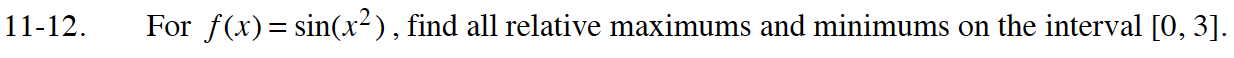### Home > CALC > Chapter 11 > Lesson 11.1.1 > Problem11-12

11-12.

For f(x) = sin(x2), find all relative maximums and minimums on the interval [0, 3]. Homework Help ✎$f^\prime(x)=2x\cos(x^2)=0$

$2x=0\text{ or }\cos(x^2)=0$

Continue to solve for x. Then determine the corresponding y-values.